# SSAT Upper Level Math : How to find the frequency of an event

## Example Questions

### Example Question #1 : Frequencies

In a small school, ninth grade comprises 54 students, including 22 boys. Each student is assigned to one of two home rooms - those of Mr. Brooks and Mrs. Kelly. The boys and the girls are both divided evenly between the home rooms.

According to the charter, two of the student council representatives from the ninth grade will be selected by choosing one at random from each home room. What is the probability that both representatives selected will be girls?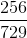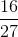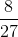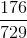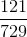Explanation:

22 out of the 54 students are boys, so 32 are girls. Also, since the boys and the girls are split evenly between the home rooms, each home room has 11 boys and 16 girls for a total of 27 students.

This means that the probability of a randomly selected student from Mr. Brooks's home room being a girl is; the probability is the same for Mrs. Kelly's homeroom. By the multiplication principle, the probability of both students being girls is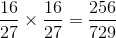### Example Question #1 : How To Find The Frequency Of An Event

Three fair six-sided dice are rolled, and the numbers are multiplied. What are the odds against rolling an odd product?

8 to 1

Even

7 to 1

4 to 1

2 to 1

7 to 1

Explanation:

Three whole numbers have an odd product if and only if all three factors are odd. Therefore, for three dice to show an odd product, all three dice must show odd numbers.

The probability of a die showing an odd number is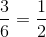, so the probability of all three dice showing odd numbers - equivalently, the probability of an odd product - is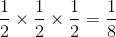. This translates to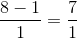or 7 to 1 odds against an odd product.

### Example Question #2 : How To Find The Frequency Of An Event

Three fair six-sided dice are rolled, and the numbers are added. What is the probability that the sum will be 16 or greater?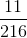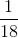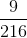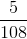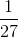Explanation:

There are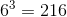outcomes for the roll of three six-sided dice. Of these outcomes, the following are the ways to roll a 16 or greater: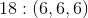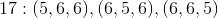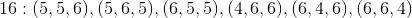This is 10 rolls with the desired outcome out of a possible 216, which translates to a probability of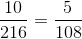### Example Question #3 : How To Find The Frequency Of An Event

The aces, jacks, kings, and queens are removed from a red-backed deck of standard playing cards; the same is done with a blue-backed deck. One card is removed at random from each deck; the numbers are multiplied together. What are the odds of the product being odd?

3 to 1 against

5 to 4 against

Even

7 to 2 against

The correct answer is not given among the other responses.

The correct answer is not given among the other responses.

Explanation:

Two whole numbers have an odd product if and only if both factors are odd.

After the aces and face cards are removed from each deck, there are nine ranks left - the twos through tens - each of which are represented by four cards and each of which are therefore equally probable in each deck. The probability of drawing an odd card from the red deck is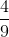, and the same holds for the blue deck. The probability of drawing two odd cards  - equivalently, the probability of an odd product - is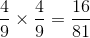; this translates to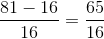or 65 to 16 odds against this occurrence. This answer is not among the responses.

### Example Question #4 : How To Find The Frequency Of An Event

In a small school, ninth grade comprises 50 students, including 18 boys. Each student is assigned to one of two home rooms - those of Mr. Jones and Mrs. Wilson. The boys and the girls are both divided evenly between the home rooms.

According to the charter, two of the student council representatives from the ninth grade will be selected by choosing one at random from each home room. What are the odds of one boy and one girl being selected?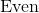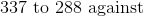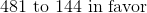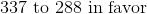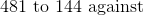Explanation:

18 out of the 50 students are boys, so 32 are girls. Also, since the boys and the girls are split evenly between the home rooms, each home room has 9 boys and 16 girls for a total of 25 students.

This means that the probability of a randomly selected student from Mr. Jones's home room being a boy is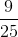, and that of the student being a girl is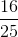. These probabilities are the same for Mrs. Wilson's class.

Therefore, the probability of one boy being selected from Mr. Jones's class and one girl being selected from Mrs. Wilson's class is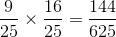; the probability of the reverse event is the same. Therefore, the probability of one boy and one girl being selected is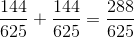The odds against this happening are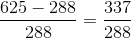- that is, 337 to 288.

### Example Question #3 : How To Find The Frequency Of An Event

If Bob rolled 3 fair six sided dice, what is the probability of NOT rolling a sum of 3?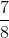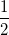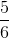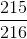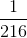Explanation:

The only possible combination to roll a sum of 3 is by rolling three 1s.  The probability of rolling any side of a die is.

The odds of rolling three 1s are: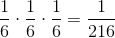Therefore, the probability of NOT rolling three 1s is: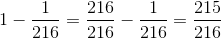### All SSAT Upper Level Math Resources